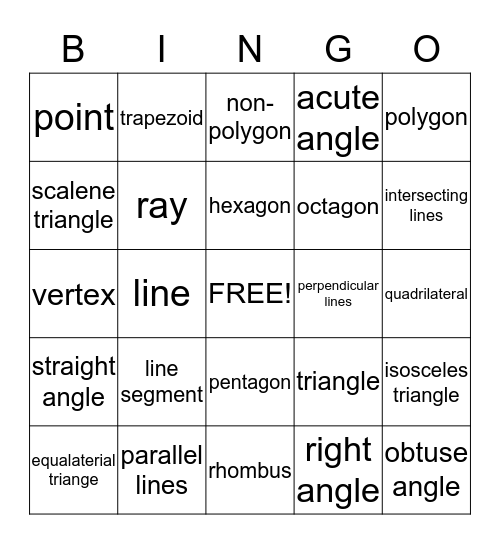# Geometry BingoThis bingo card has a free space and 24 words: line segment, parallel lines, hexagon, equalaterial triange, polygon, point, scalene triangle, trapezoid, perpendicular lines, pentagon, octagon, vertex, triangle, isosceles triangle, ray, right angle, quadrilateral, non-polygon, intersecting lines, line, obtuse angle, straight angle, acute angle and rhombus.

## Play Online